NCERT Solutions for Class 8 Maths Chapter 6 Squares and Square Roots Ex 6.3

# NCERT Solutions for Class 8 Maths Chapter 6 Squares and Square Roots Ex 6.3

## NCERT Solutions for Class 8 Maths Chapter 6 Squares and Square Roots Ex 6.3

NCERT Solutions for Class 8 Maths Chapter 6 Squares and Square Roots Ex 6.3 are the part of NCERT Solutions for Class 8 Maths. Here you can find the NCERT Solutions for Class 8 Maths Chapter 6 Squares and Square Roots Ex 6.3.

### Ex 6.3 Class 8 Maths Question 1.

What could be the possible ‘one’s’ digits of the square root of each of the following numbers?
(i) 9801
(ii) 99856
(iii) 998001
(iv) 657666025

Solution:
(i) The one’s digit in the square root of 9801 is either 1 or 9.
(ii) The one’s digit in the square root of 99856 is either 4 or 6.
(iii) The one’s digit in the square root of 998001 is either 1 or 9.
(iv) The one’s digit in the square root of 657666025 is 5.

### Ex 6.3 Class 8 Maths Question 2.

Without doing any calculation, find the numbers which are surely not perfect squares.
(i) 153
(ii) 257
(iii) 408
(iv) 441

Solution:
We know that the numbers which end with 2, 3, 7 or 8 are not perfect squares.
(i) The number 153 is not a perfect square. (It ends with 3)
(ii) The number 257 is not a perfect square number. (It ends with 7)
(iii) The number 408 is not a perfect square number. (It ends with 8)
(iv) The number 441 is a perfect square.

### Ex 6.3 Class 8 Maths Question 3.

Find the square roots of 100 and 169 by the method of repeated subtraction.

Solution:
Using the method of repeated subtraction of consecutive odd numbers, we have
(i) 100 – 1 = 99, 99 – 3 = 96, 96 – 5 = 91, 91 – 7 = 84, 84 – 9 = 75, 75 – 11 = 64, 64 – 13 = 51, 51 – 15 = 36, 36 – 17 = 19, 19 – 19 = 0
(Ten times repetition)
Thus, √100 = 10

(ii) 169 – 1 = 168, 168 – 3 = 165, 165 – 5 = 160, 160 – 7 = 153, 153 – 9 = 144, 144 – 11 = 133, 133 – 13 = 120, 120 – 15 = 105, 105 – 17 = 88, 88 – 19 = 69, 69 – 21 = 48, 48 – 23 = 25, 25 – 25 = 0
(Thirteen times repetition)
Thus, √169 = 13

### Ex 6.3 Class 8 Maths Question 4.

Find the square roots of the following numbers by the prime factorisation method.
(i) 729
(ii) 400
(iii) 1764
(iv) 4096
(v) 7744
(vi) 9604
(vii) 5929
(viii) 9216
(ix) 529
(x) 8100

Solution:
(i) Let us find the prime factorisation of 729.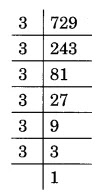729 = 3 × 3 × 3 × 3 × 3 × 3 = 32 × 32 × 32
√729 = 3 × 3 × 3 = 27

(ii) Let us find the prime factorisation of 400.400 = 2 × 2 × 2 × 2 × 5 × 5 = 22 × 22 × 52
√400 = 2 × 2 × 5 = 20

(iii) Let us find the prime factorisation of 1764.

(iv) Let us find the prime factorisation of 4096.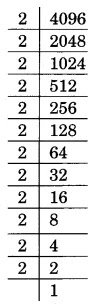4096 = 2 × 2 × 2 × 2 × 2 × 2 × 2 × 2 × 2 × 2 × 2 × 2
= 22 × 22 × 22 × 22 × 22 × 22
√4096 = 2 × 2 × 2 × 2 × 2 × 2 = 64

(v) Let us find the prime factorisation of 7744.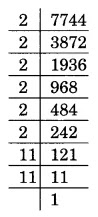7744 = 2 × 2 × 2 × 2 × 2 × 2 × 11 × 11
= 22 × 22 × 22 × 112
√7744 = 2 × 2 × 2 × 11 = 88

(vi) Let us find the prime factorisation of 9604.

(vii) Let us find the prime factorisation of 5929.

(viii) Let us find the prime factorisation of 9216.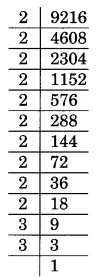9216 = 2 × 2 × 2 × 2 × 2 × 2 ×2 × 2 × 2 × 2 × 3 × 3
= 22 × 22 × 22 × 22 × 22 × 32
√9216 = 2 × 2 × 2 × 2 × 2 × 3 = 96

(ix) Let us find the prime factorisation of 529.

(x) Let us find the prime factorisation of 8100.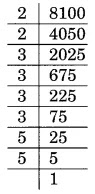8100 = 2 × 2 × 3 × 3 × 3 × 3 × 5 × 5 = 22 × 32 × 32 × 52
√8100 = 2 × 3 × 3 × 5 = 90

### Ex 6.3 Class 8 Maths Question 5.

For each of the following numbers, find the smallest whole number by which it should be multiplied so as to get a perfect square number. Also, find the square root of the square number so obtained.
(i) 252
(ii) 180
(iii) 1008
(iv) 2028
(v) 1458
(vi) 768

Solution:
(i) Let us find the prime factorisation of 252.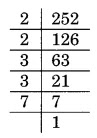252 = 2 × 2 × 3 × 3 × 7
Here, the prime factorisation is not in pair. The factor 7 has no pair.
Thus, 7 is the smallest whole number by which the given number is to be multiplied to get a perfect square number.
The new square number = 252 × 7 = 1764
Square root of 1764 = √1764 = 2 × 3 × 7 = 42

(ii) Let us find the prime factorisation of 180.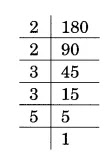180 = 2 × 2 × 3 × 3 × 5
Here, the prime factorisation is not in pair. The factor 5 has no pair.
Thus, 5 is the smallest whole number by which the given number is to be multiplied to get a perfect square number.
The new square number = 180 × 5 = 900
The square root of 900 = √900 = 2 × 3 × 5 = 30

(iii) Let us find the prime factorisation of 1008.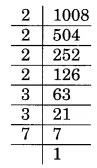1008 = 2 × 2 × 2 × 2 × 3 × 3 × 7
Here, the prime factorisation is not in pair. The factor 7 has no pair.
Thus, 7 is the smallest whole number by which the given number is to be multiplied to get a perfect square number.
The new square number = 1008 × 7 = 7056
Square root of 7056 = √7056 = 2 × 2 × 3 × 7 = 84

(iv) Let us find the prime factorisation of 2028.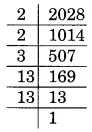2028 = 2 × 2 × 3 × 13 × 13
Here, the prime factorisation is not in pair. The factor 3 has no pair.
Thus, 3 is the smallest whole number by which the given number is to be multiplied to get a perfect square number.
The new square number = 2028 × 3 = 6084
Square root of 6084 = √6084 = 2 × 13 × 3 = 78

(v) Let us find the prime factorisation of 1458.1458 = 2 × 3 × 3 × 3 × 3 × 3 × 3
Here, the prime factorisation is not in pair. The factor 2 has no pair.
Thus, 2 is the smallest whole number by which the given number is to be multiplied to get a perfect square number.
The new square number = 1458 × 2 = 2916
Square root of 2916 = √2916 = 3 × 3 × 3 × 2 = 54

(vi) Let us find the prime factorisation of 768.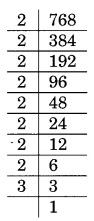768 = 2 × 2 × 2 × 2 × 2 × 2 × 2 × 2 × 3
Here, the prime factorisation is not in pair. The factor 3 has no pair.
Thus, 3 is the smallest whole number by which the given number is to be multiplied to get a perfect square number.
The new square number = 768 × 3 = 2304
Square root of 2304 = √2304 = 2 × 2 × 2 × 2 × 3 = 48

### Ex 6.3 Class 8 Maths Question 6.

For each of the following numbers, find the smallest whole number by which it should be divided so as to get a perfect square. Also, find the square root of the square number so obtained.
(i) 252
(ii) 2925
(iii) 396
(iv) 2645
(v) 2800
(vi) 1620

Solution:
(i) Let us find the prime factorisation of 252.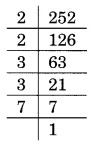252 = 2 × 2 × 3 × 3 × 7
Here, the prime factorisation is not in pair. The factor 7 has no pair.
Thus, 7 is the smallest whole number by which the given number is to be divided to get a perfect square number.
The new square number = 252 ÷ 7 = 36
Thus, √36 = 2 × 3 = 6

(ii) Let us find the prime factorisation of 2925.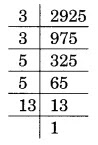2925 = 3 × 3 × 5 × 5 × 13
Here, the prime factorisation is not in pair. The factor 13 has no pair.
Thus, 13 is the smallest whole number by which the given number is to be divided to get a perfect square number.
The new square number = 2925 ÷ 13 = 225
Thus, √225 = 3 × 5 = 15

(iii) Let us find the prime factorisation of 396.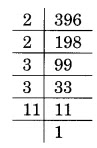396 = 2 × 2 × 3 × 3 × 11
Here, the prime factorisation is not in pair. The factor 11 has no pair.
Thus, 11 is the smallest whole number by which the given number is to be divided to get a perfect square number.
The new square number = 396 ÷ 11 = 36
Thus, √36 = 2 × 3 = 6

(iv) Let us find the prime factorisation of 2645.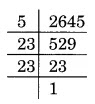2645 = 5 × 23 × 23
Here, the prime factorisation is not in pair. The factor 5 has no pair.
Thus, 5 is the smallest whole number by which the given number is to be divided to get a perfect square number.
The new square number = 2645 ÷ 5 = 529
Thus, √529 = 23

(v) Let us find the prime factorisation of 2800.2800 = 2 × 2 × 2 × 2 × 5 × 5 × 7
Here, the prime factorisation is not in pair. The factor 7 has no pair.
Thus, 7 is the smallest whole number by which the given number is to be divided to get a perfect square number.
The new square number = 2800 ÷ 7 = 400
Thus, √400 = 2 × 2 × 5 = 20

(vi) Let us find the prime factorisation of 1620.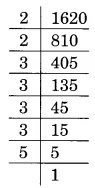1620 = 2 × 2 × 3 × 3 × 3 × 3 × 5
Here, the prime factorisation is not in pair. The factor 5 has no pair.
Thus, 5 is the smallest whole number by which the given number is to be divided to get a perfect square number.
The new square number = 1620 ÷ 5 = 324
Thus, √324 = 2 × 3 × 3 = 18

### Ex 6.3 Class 8 Maths Question 7.

The students of Class VIII of a school donated Rs 2401 in all, for Prime Minister’s National Relief Fund. Each student donated as many rupees as the number of students in the class. Find the number of students in the class.

Solution:
Let x be the number of students in the class. Therefore, total money donated by students

Hence, the number of the students in the class is 49.

### Ex 6.3 Class 8 Maths Question 8.

2025 plants are to be planted in a garden in such a way that each row contains as many plants as the number of rows. Find the number of rows and the number of plants in each row.

Solution:
Let x be the number of rows and x be the number of plants in each row.
The total number of plants = x × x = x2
But is it given as 2025.

Hence, the number of rows is 45 and the number of plants in each row is also 45.

### Ex 6.3 Class 8 Maths Question 9.

Find the smallest square number that is divisible by each of the numbers 4, 9 and 10.

Solution:
The smallest number divisible by each of the numbers 4, 9 and 10 is their LCM, which is (2 × 2 × 9 × 5), i.e., 180.
Now, 180 = 2 × 2 × 3 × 3 × 5

This in not a square number because 5 is not in pair.
To make it a perfect square, it must be multiplied by 5.
Required square number = 180 × 5 = 900

### Ex 6.3 Class 8 Maths Question 10.

Find the smallest square number that is divisible by each of the numbers 8, 15 and 20.

Solution:
The smallest number divisible by each of the numbers 8, 15 and 20 is their LCM, which is (2 × 2 × 5 × 2 × 3), i.e., 120.
Now, 120 = 2 × 2 × 2 × 3 × 5
To make it a perfect square, it must be multiplied by
2 × 3 × 5, i.e., 30.

Required square number = 120 × 30 = 3600

You can also like these:

NCERT Solutions for Maths Class 9

NCERT Solutions for Maths Class 10

NCERT Solutions for Maths Class 11

NCERT Solutions for Maths Class 12